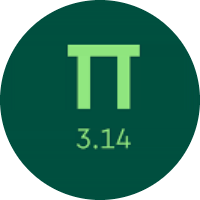# Learning the Linear Equations

In this quiz, you will get to know about Linear Equations. You will find practice problems on finding the slope of a line, and writing equations of the lines by different methods. Also, you will learn about the slope of perpendicular and parallel lines.

Start Quiz

Find the slope of the line that passes through (10, 8) and (7, 7).

1/3

2/3

1/4

2/5

A line has a slope of 8 and includes the points (4,a) and (5,7). What is the value of a?

-2

-1

3

1

Find the slope of the line y = (9/10)x + (3/10).

3/10

9

3

9/10

Find the y-intercept of the line y = (1/3)x - 1.

-1

1/3

1

2

There is a line whose slope is 6 and the y-intercept is -7. What is its equation in slope-intercept form?

y = 7x - 6

y = -7x + 6

y = 6x - 7

x = 6y - 7

A line has a slope of 5 and passes through the point (3,5). What is its equation in slope-intercept form?

y = 3x - 5

y = 5x - 15

y = 5x - 3

y = 5x - 10

A line passes through the points (0, 4) and (3, 10). What is its equation in slope-intercept form?

y = ##2##x + ##4##

3 -1 2 -2 4

Rewrite the following equation in slope-intercept form.

2x + 17y = -12

y = (-2/17)x - 12/17

y = (-2)x - 12

y = (-2/17)x - 5/17

y = (-6/17)x - 12/17

Line s has a slope of 3/5. Line s is perpendicular to line p. What will be the slope of line p?

3/5

5/3

-3/5

-5/3

Line x has a slope of 5/6. Line y also has the same slope. Both of the lines are:

Perpendicular

Parallel

Neither

Quiz/Test Summary
Title: Learning the Linear Equations
Questions: 10
Contributed by: